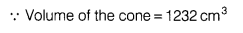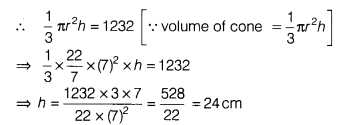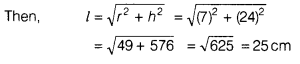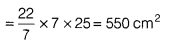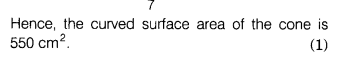# The volume of a right circular cone is 1232m3. If the diameter of the base is 14cm

The volume of a right circular cone is 1232m3. If the diameter of the base is 14cm, then find [Take π =22/7]
(i) height of the cone.
(ii) slant height of the cone.
(iii) curved surface area of the cone

Given, diameter of the base = 14cm